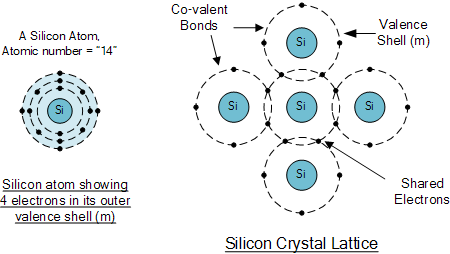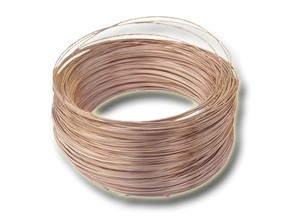×#### Thank you for registering.

One of our academic counsellors will contact you within 1 working day.

Click to Chat

1800-1023-196

+91-120-4616500

CART 0

• 0

MY CART (5)

Use Coupon: CART20 and get 20% off on all online Study Material

ITEM
DETAILS
MRP
DISCOUNT
FINAL PRICE
Total Price: Rs.

There are no items in this cart.
Continue Shopping• Complete Physics Course - Class 11
• OFFERED PRICE: Rs. 2,968
• View Details

```Temperature Dependence of Resistivity

Resistivity

Temperature Dependence of Resistivity

Variation of Resistivity in Conductors

Variation of Resistivity in Semiconductors

Variation of Resistivity in Insulators

Superconductors

Other Materials

Factors affecting resistivity

Summary

Resistivity

Resistivity is known as specific electrical resistance or volume resistivity. It can be defined as the intrinsic property of a given material that shows how it opposes the flow of current. It can also be defined as the resistance offered by a conductor having unit length and unit area of cross section. So it does not depend upon the length and area of cross section of a material. But the resistance of a material depends upon the length and area of cross section of the material. The resistivity is expressed as ρ = R A/L, where R is the resistance in ohms, A is the area of cross section in square meters and L is the length in meters. The unit of resistivity is ohm meter.

Temperature Dependence of Resistivity

The resistivity of materials depend on the temperature. ρt = ρ0 [1 + α (T – T0) is the equation that shows the relation between the temperature and the resistivity of a material. In the equation ρ0 is the resistivity at a standard temperature, ρt is the resistivity at t0 C, T0  is the reference temperature and α is the temperature co – efficient of resistivity.

Variation of Resistivity in Conductors

We know that current is the movement of free electrons from one atom to the other when there is a potential difference. In conductors no forbidden gap is present between the conduction band and valence band. In many cases both the bands overlap each other. The valence electrons are loosely bound to the nucleus in conductors. Usually metals or conductors have low ionization energy and so they tend to lose electrons very easily. When an electric current is applied the delocalized electrons are free to move within the structure. This is the case that happens in normal temperature.

When the temperature increases the vibrations of the metal ions in the lattice structure increases. The atoms starts to vibrate with higher amplitude. These vibrations in turn causes frequent collisions between the free electrons and the other electrons. Each collision drain out some energy of the free electrons and causing them unable to move. Thus it restricts the movement of the delocalized electrons. When the collision happens the drift velocity of the electrons decreases. This means that the resistivity of the metal increases and thus current flow in the metal is decreased. The resistivity increases means that the conductivity of the material decreases.

For metals or conductors, it is said that they have a positive temperature co – efficient. The value α is positive. For most of the metals, the resistivity increases linearly with increase in temperature for a range of 500K. Examples for positive temperature co – efficient include, silver, copper, gold etc.Temperature dependence on resistivity for metals

Variation of Resistivity in Semiconductors

Silicon is a semiconductor. In semiconductors the forbidden gap between the conduction band and the valence band is small. At 0K, the valence band is completely filled and the conduction band may be empty. But when a small amount of energy is applied, the electrons easily moves to the conduction band. Silicon is an example for semiconductor. Under normal circumstances silicon act as a poor conductor. Each silicon atom is bonded to 4 other silicon atoms. The bonds between these atoms are co valent bonds where the electrons are in fixed positons. So at 0K, the electrons does not move within the lattice structure.When the temperature in increased the forbidden gap between the two bands becomes very less and the electrons move from the valence band to the conduction band. Thus some electrons from the co valent bonds between the Si atoms are free to move within the structure. This increases the conductivity of the material. The conductivity increases means the resistivity decreases. Thus when the temperature is increased in a semiconductor, the density of the charge carriers also increases and the resistivity decreases. For semiconductors it is said that they have a negative temperature co – efficient. So the value of temperature co –efficient of resistivity, α is negative.

The curve is non - linear for a wide range of temperature.Temperature dependence on resistivity for semiconductors

Variation of Resistivity in Insulators

In insulators the forbidden energy gap between the conduction band and the valence band is high. The valence band is completely filled with the electrons. The forbidden gap between the two bands will be more than 3 e V. Thus it requires a high amount to energy for the valence electron to move to the conduction band. Diamond is an example for insulator. Here all the valence electrons are involved in the co valent bond formation and conduction does not happen. The electrons are tightly bound to the nucleus.

When the temperature is increased, the atoms of the material vibrate and it makes the valence electrons present in the valence band to shift to the conduction band. This in turn increases the conductivity of the material. When the conductivity of the material increases, it means that the resistivity decreases and so the current flow increases. Thus some insulators at room temperatures changes to conductors at high temperature. For insulators they have a negative temperature co – efficient. So the value of temperature co –efficient of resistivity, α is negative.Conductors and insulators

Superconductors

We know that when an electric current is passed through a conductors some energy is lost in the form of heat. The amount of energy loss varies depending on the resistance of the material. In 1911 some scientists cooled a sample of mercury down to 4.2° above absolute zero. Thus the resistance of the material changed to zero. Thus the first super conductor was discovered. Thus the scientists found that in some circumstances some materials do not show any resistance. The materials with zero resistance are called super conductors. At zero resistance the materials conducts current without any loss of energy. When the temperature of such materials are decreased, the free electrons stop colliding with the positive ions and thus it offers zero resistance. The temperature at which the resistance falls to zero is called Critical Temperature.

When the superconductor is placed in the magnetic field, the magnetic field bends around the material as it does not allow the magnetic field to pass through them. When the intensity of the magnetic field is increased, at a certain point the field is able to penetrate through the super conductor and thus its behavior is destroyed.

Consider an electric current is passed through the superconductor. Suppose the density of the current is increased, at a particular value of current density, it loses its superconductivity and finally behaves like a normal material. The current density above which the material loses its superconductivity is called the critical current density. High temperature, high magnetic field and high current density will destroy the behavior of superconductivity of a material. Now a days these materials are used in MRI machines.

Other Materials

The resistivity of materials like nichrome, manganin and constantan does not depend much on temperature and show a very low dependence. Hence these materials are used in wire bound standard resistors as the variation in the value of resistance is negligible when the temperature changes.Manganin
Constantan

Factors affecting resistivity

We know that the resistivity, ρ = m/n e2ԏ, where e is the charge on an electron, ԏ is the average time taken between each collision or the relaxation time of electrons and m is the mass of the electron, n is the charge density.  Thus it shows that the resistivity depends on the number of factors like the relaxation time between the collisions and the charge density. From the above scenarios it is clear that when the temperature is increased the average speed of the electrons increases and thus more collision occurs. Thus the relaxation time between each collisions decreases.

In case of metals the charge density does not depend on temperature to a certain extent. So the other factors like ԏ is affected, which means when the temperature is increases the average time between the collisions is decreases causing the resistivity to increase.

For semiconductors and insulators, the charge density n increases when the temperature is increased. It compensates the decrease in the value of ԏ. Hence the resistivity decreases when the temperature decreases.

Summary

The resistivity is the resistance offered by a conductor having unit length and unit area of cross section. The unit of resistivity is ohm meter. The formula is ρ = RA/L, where R is the resistance in ohms, A is the area of cross section in square meters and L is the length in meters.

ρt = ρ0 [ 1 + α (T – T0) is the equation that shows the relation between the temperature and the resistivity of a material. ρ0 is the resistivity at a standard temperature, ρt is the resistivity at t0 C, T0  is the reference temperature and α is the temperature co – efficient of resistivity.

For metals or conductors, when the temperature increases and the resistivity of the metal increases and thus current flow in the metal is decreased. They have a positive temperature co – efficient. The value α is positive.

For semiconductors, when the temperature is increased the conductivity of the material is increased. It means that the resistivity of the material is decreased and so the current flow is increased. For semiconductors they have a negative temperature co – efficient. So the value of temperature co –efficient of resistivity, α is negative.

For insulators the conductivity of the material is increased, when the temperature is increased. When the conductivity of the material is increased, we know that the resistivity decreases and the current flow thus increases. So some insulators at room temperatures changes to conductors at high temperature. For insulators they have a negative temperature co – efficient. The value of temperature co –efficient of resistivity, α is negative.

The materials with zero resistance are called super conductors. The temperature at which the resistance drops to zero is called the critical temperature. High temperature, high magnetic field and high current density will loosen the property of superconductivity of a material. Mercury is an example for super conductor.

The materials like nichrome, manganin and constantan does not depend much on temperature. So the change in the resistivity of the material during temperature change is negligible.

Watch this Video for more reference

Temperature Dependence of Resistivity
```### Course Features

• 101 Video Lectures
• Revision Notes
• Previous Year Papers
• Mind Map
• Study Planner
• NCERT Solutions
• Discussion Forum
• Test paper with Video Solution